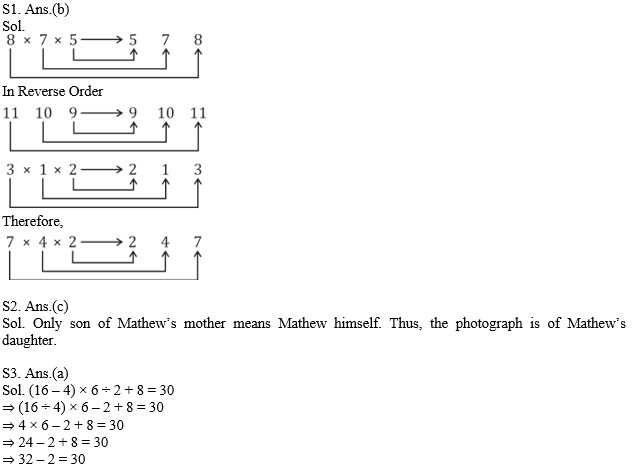The reasoning is the most scoring section in any competitive exam. It also develops or nourishes our critical thinking skills as compared to other subjects. In DSSSBKVSNVS Exam, as a student to score well in this part, you have to start your preparation now because there is negative marking as well. The more you have prepared, the better you score.

Q1. Some equations are solved on the basis of a certain system. On the same basis, find out the correct answer for the unsolved equation.
8 × 7 × 5 = 578;
11 × 10 × 9 = 91011;
3 × 1 × 2 = 213;
7 × 4 × 2 = ?

(a) 56
(b) 247
(c) 128
(d) 282

Q2. Mathew told his friend Sham, pointing to a photograph, “Her father is the only son of my mother.” The photograph is of whom?

(a) Mathew’s niece
(b) Mathew’s mother
(c) Mathew’s daughter
(d) Mathew’s sister

Q3. Which interchange of signs will make the following equation correct?
(16 – 4) × 6 ÷ 2 + 8 = 30

(a) ÷ and –
(b) 4 and 2
(c) – and +
(d) 16 and 6

Q4. A group of alphabets are given with each being assigned a number. These have to be unscrambled into a meaningful word and correct order of letters may be indicated from the given responses.(a) 2, 5, 1, 3, 4
(b) 4, 3, 5, 1, 2
(c) 5, 4, 1, 3, 2
(d) 5, 4, 2, 3, 1

Q5. Find the wrong number in the given series:
9, 14, 19, 25, 32, 40

(a) 14
(b) 25
(c) 32
(d) 9

Q6. From the given alternatives select the word which cannot be formed using the letters of the given word?
PSYCHOLOGY

(a) SCHOOL
(b) GOOD
(c) POOL
(d) HOLY

Q7. In a group of five districts Akbarpur is smaller than Fatehpur, Dhanbad is bigger than Palamu and Bara Banki is bigger than Fatehpur but not as big as Palamu. Which district is the biggest?

(a) Akbarpur
(b) Fatehpur
(d) Palamu

Q8. Select the correct combination of mathematical signs to replace * signs and to balance the given equation.
18 * 6 * 3 * 12 * 24

(a) ÷ – = ×
(b) × ÷ – =
(c) + ÷ × =
(d) × = ÷ +

Q9. If TALENT is written as LATENT, how EXOTIC can be written in that code?

(a) OXOTIC
(b) TEXTIC
(c) OXETIC
(d) EXOTIC

Directions (10): In the following question, select the odd word from the given alternatives.
Q10.

(a) Square
(b) Rectangle
(c) Cylinder
(d) Triangle

Solutions